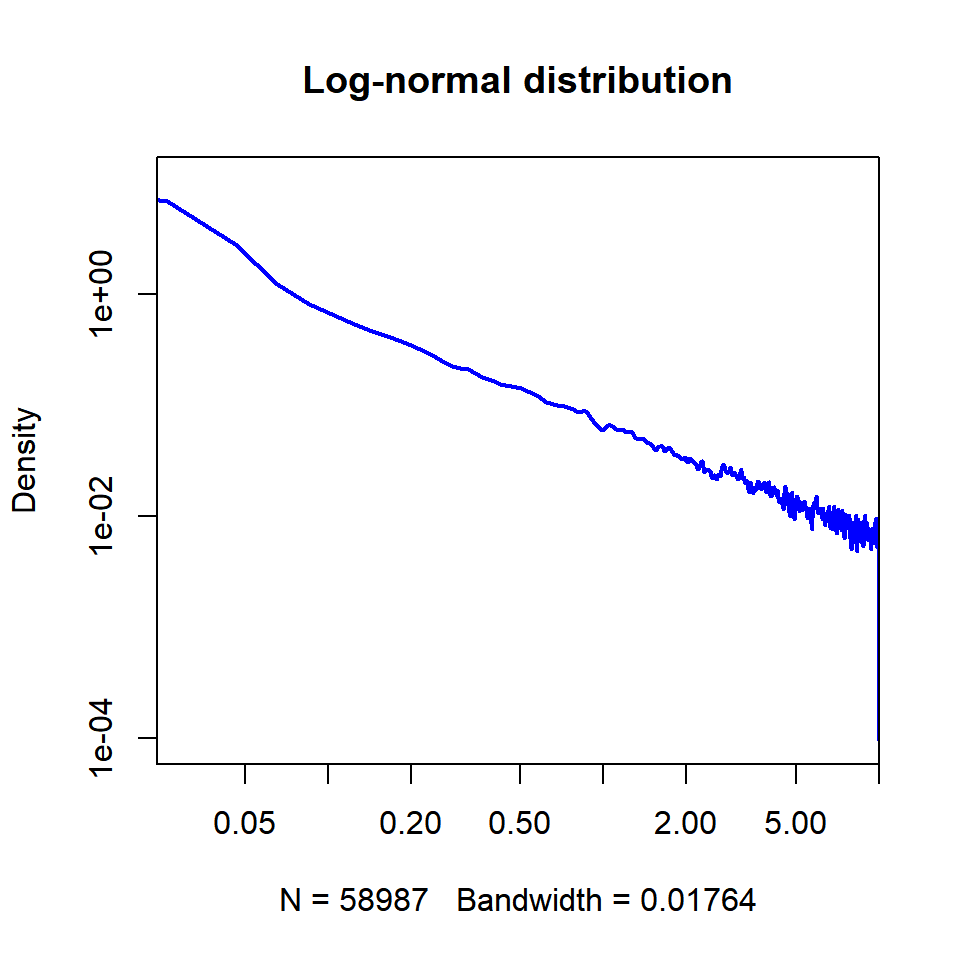.— output: html_document: css: custom.css —

### POWER LAW DISTRIBUTION:

From Wolfram alpha,

The pdf of the power law is

$f_X(x) =C x^\alpha, x \in [x_0, x_1]$ and the normaliztion constant can be determined by considering that $$f_X(x)$$ has to integrate to 1:

$\int_{x_0}^{x_1} f_X(x)dx= C \frac{[x^{\alpha+1}]_{x_0}^{x_1}}{\alpha+1}=1$ and hence,

$C=\frac{\alpha+1}{x_1^{\alpha+1}- x_0^{\alpha+1}}.$

However, notice that in other sources, the negative value of alpha is explicit in the equations. For instance:

$p(x)=\frac{\alpha - 1}{x_{\mathrm{min}}}\left(\frac{x}{x_{\mathrm{min}}} \right)^{-\alpha}$

From pag 4 of this article by M. E. J. Newman, the majority of power law distributions occurring in nature $$2\leq \alpha \leq 3.$$

The cumulative probability distribution is

$F_X(x) = \Pr(X <x)=\int_{x_0}^x C x^\alpha dx =\frac{C}{\alpha+1}\left(x^{\alpha+1}-x_0^{\alpha+1 }\right)$

If $$Y\sim U[0,1]$$ and by the probability integral transform,

$y\equiv \frac{C}{\alpha+1} \left(x^{\alpha+1}-x_0^{\alpha+1} \right)$

So $$y=g(x)$$ with inverse

$X = \left( \frac{\alpha+1}{C}y + x_0^{\alpha+1} \right)^{1/{\alpha+1}}$

and replacing $$C$$

$X = \left[ (x_1^{\alpha+1}-x_0^{\alpha+1})y + x_0^{\alpha+1} \right]^{1/{\alpha+1}}$

and $$X\sim L(x),$$ i.e. it is distributed following a power law.

Note that it is different from page 3 of this document.

Plotting a power law distribution in log-log will result in a line:

$f_X(x)= C\, x^{-\alpha}$

$\log(f_X(x))=\log C + -\alpha\, \log x$

with slope $$-\alpha$$

In R

x1 = 5
x0 = 0.1     # It can't be zero; otherwise X^0^(neg) is 1/0.
alpha = -2.5  # It has to be negative.
set.seed(123)
y = runif(1e5)
x = ((x1^(alpha+1) - x0^(alpha+1))*y + x0^(alpha+1))^(1/(alpha+1))
summary(x)
##    Min. 1st Qu.  Median    Mean 3rd Qu.    Max.
##  0.1000  0.1211  0.1579  0.2579  0.2501  4.9910
hist(x, xlim = c(0,1), ylim = c(0,12), border=F, col=rgb(0,0,0.8,.5),
breaks=200,
cex.main=.7, cex.lab=.7, prob=T,
main="Power law")
lines(density(x), col=4, lwd=1)
lines(density(x, adjust=2), lty="dotted", col="turquoise", lwd=2)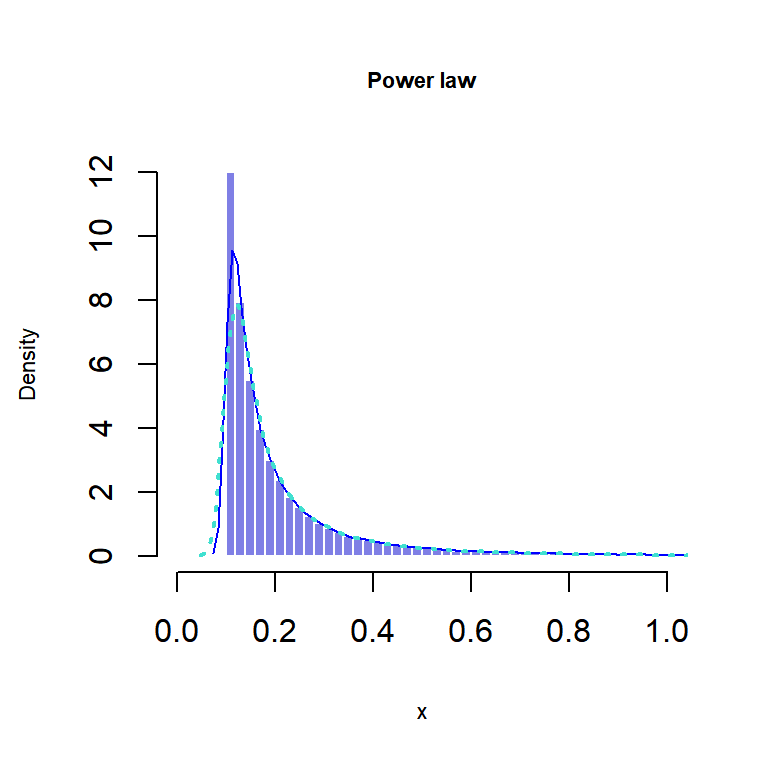h = hist(x, prob=T, breaks=40, plot=F)
plot(h\$count, log="xy", type='l', lwd=1, lend=2, xlab="", ylab="", main="Density in logarithmic scale")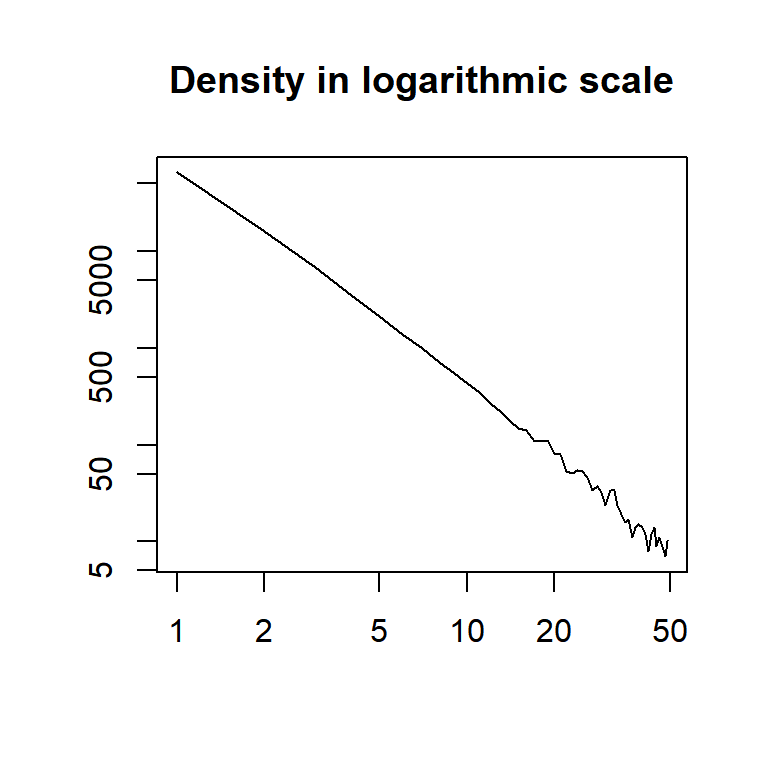The number of samples in the bins becomes small towards the right of the plot, leading to statistical fluctuations, and the noisy right tails.

If we hadn’t generated these data synthetically, and were looking at $$x$$ as empirical data from an experiment, we could try to fit a power law distribution with the package igraph and the functionpower.law.fit().

##### Power-law distributions:
1. Zeta distribution
2. Lévy distribution
3. Lévy skew alpha-stable distribution
4. Pareto distribution
5. Zipf-Mandelbrot law
6. Infinitely divisible distribution

###### Pareto distribution:

$f_X(x) = \frac{\alpha \, x^\alpha_m}{x^{\alpha+1}}$

with support $$x\in [x_m, +\infty),$$ and $$x_m>0$$ and $$\alpha>0$$ corresponding to shape parameters. The parameter $$\alpha$$ is called the tail index, or the Pareto index when discussing distribution of wealth.

When plotted on linear axes, the distribution assumes the familiar J-shaped curve which approaches each of the orthogonal axes asymptotically. All segments of the curve are self-similar (subject to appropriate scaling factors). When plotted in a log-log plot, the distribution is represented by a straight line.

#### Subexponential distributions:

There are three important subclasses of heavy-tailed distributions: the fat-tailed distributions, the long-tailed distributions and the subexponential distributions. In practice, all commonly used heavy-tailed distributions belong to the subexponential class.

##### Heavy-tailed distributions:

All commonly used heavy-tailed distributions are subexponential.

1. Pareto
2. Log-normal
3. Lévy distribution
4. Weibull distribution
5. Burr distribution
6. Log-gamma distribution
7. Log-Cauchy distribution (“super heavy tail”)
8. Cauchy
9. Stable distributions (except for the Gaussian) - Cauchy, Lévy and Lommel
10. Student t distribution

##### Subexponential distributions:

From these CalTech notes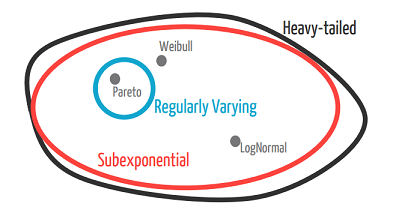Exponential distributions are not subexponential!

x1 = 5
x0 = 0.07     # It can't be zero; otherwise X^0^(neg) is 1/0.
alpha = -2.5  # It has to be negative.

set.seed(123)
y = runif(1e5)
x = ((x1^(alpha+1) - x0^(alpha+1))*y + x0^(alpha+1))^(1/(alpha+1))

par(mfrow = c(1,2))

hist(x, xlim = c(0,1), border=F, col=2, breaks=80,
cex.main=.7, cex.lab=.7, ylim= c(0,12000),
main="Power law")
abline(v=.4, lty=2)

y = rexp(1e5, rate = 15)
hist(y, breaks=20, xlim = c(0,1), ylim= c(0,12000),border=F, col=2, cex.main=.7, cex.lab=.7,
main="Exponential")
abline(v=.4, lty=2)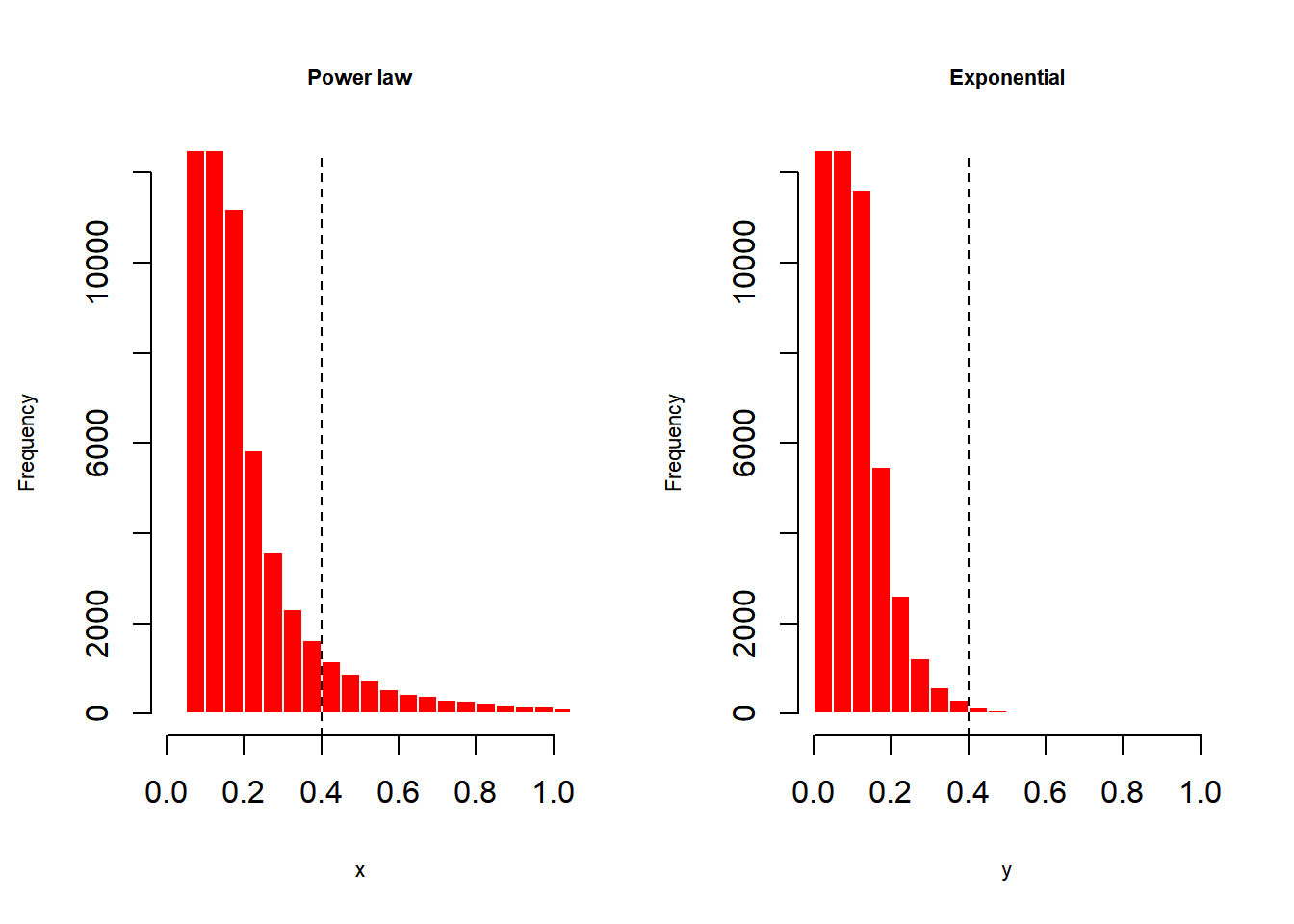These distributions can be intuitively approximated through these heuristics:

1. The principle of the single big jump

2. Many mice and a few elephants

3. The catastophe principle (versus the conspiracy principle of light tailed distributions).

E.g. the sum up the estimated cost of the damages from each hurricane during a given year is unexpectedly large. It is probably not because there were a lot of hurricanes that caused larger than typical amounts of damage.The catastrophe principle for heavy-tailed distributions tells us that the most likely reason that the sum is unexpectedly large is because there was one hurricane that caused an extremely large amount of damage. In fact, we should expect that there was one hurricane that caused nearly all of the damage caused during the year, i.e., there was a catastrophe.

Mathematically,

A distribution $$F$$ on the positive half-line is subexponential if

$\overline{F^{*n}}(x) \sim n\,\bar F(x), x \to \infty$

The probabilistic interpretation of this is that, for a sum of $$\displaystyle n$$ independent random variables $$\displaystyle X_{1},\ldots ,X_{n}$$ with common distribution $$\displaystyle F$$,

$\displaystyle \Pr[X_{1}+\cdots +X_{n}>x]\sim \Pr[\max(X_{1},\ldots ,X_{n})>x]\quad {\text{as }}x\to \infty .$

#### CONNECTION POWERLAW AND LOGNORMAL:

Not all highly right-skewed distributions follow the power law. The log-normal distribution can have very similar plots.

From this source

The log-normal is seen as a result of multiplying random variables, as much as adding random variables result in a normal distribution (central limit theorem).

The log-normal (or lognormal) distribution is a continuous probability distribution of a random variable whose logarithm is normally distributed. It is a subexponential distribution, $$\text{Lognormal}(\mu,\sigma^2)$$ with pdf,

$f_X(x) =\frac{1}{x\sqrt{2\pi \sigma^2}}\,\exp\left[{-\frac{(\ln x-\mu)^2}{2\sigma^2}}\right]$

Derivation from the normal:

$f_X(x) =\frac{1}{\sqrt{2\pi \sigma^2}}\,\exp\left[{-\frac{( x-\mu)^2}{2\sigma^2}}\right]$

If we look at it as a change of variables, the Jacobian will account for $$\frac{1}{x}$$ as we apply the chain rule, $$\frac{d}{dx}\ln x= \frac{1}{x}.$$

In base $$10$$ the pdf is

$f_X(x) =\frac{\log_{10}(e)}{10^x\sqrt{2\pi \sigma^2}}\,\exp\left[{-\frac{( x-\mu)^2}{2\sigma^2}}\right]$

If we start off with

$f_X(x) =\frac{1}{y\ln(10)\sqrt{2\pi \sigma^2}}\,\exp\left[{-\frac{( \log_{10}(y)-\mu)^2}{2\sigma^2}}\right]$

because $$d/dx \log_{10}(y) = \frac{1}{y \ln(10)}.$$ Now, since $$\ln(10)=\frac{\log_{10}(10)}{\log_{10}(e)}=\frac{1}{\log_{10}e},$$

$f_X(x) =\frac{\log_{10}(e)}{y\sqrt{2\pi \sigma^2}}\,\exp\left[{-\frac{( \log_{10}(y)-\mu)^2}{2\sigma^2}}\right]$

If we substitute $$y = 10^x,$$

$f_X(x) =\frac{\log_{10}(e)}{10^x\sqrt{2\pi \sigma^2}}\,\exp\left[{-\frac{( x-\mu)^2}{2\sigma^2}}\right]$

In the log-log plots, the log-normal will assume a shifted and inverted parabola:

$\log\left(f_X(x)\right) =\log\left\{\frac{\exp\left[{-\frac{(\ln x-\mu)^2}{2\sigma^2}}\right]}{x\sqrt{2\pi \sigma^2}}\right\}$

\begin{align} \log\left(f_X(x)\right) &=\log\left\{\exp\left[{-\frac{(\ln x-\mu)^2}{2\sigma^2}}\right]\right\}- \log \left\{x\sqrt{2\pi \sigma^2}\right\}\\[2ex] &=-\frac{(\ln x-\mu)^2}{2\sigma^2}- \log \left\{x\sqrt{2\pi \sigma^2}\right\} \end{align}

set.seed(1)
x = rlnorm(1e5)
x = x[x < 10]
plot(density(x), log="xy", type="l", xlim=c(.03, 8), col = 4, lwd = 2,
main="Log-normal distribution")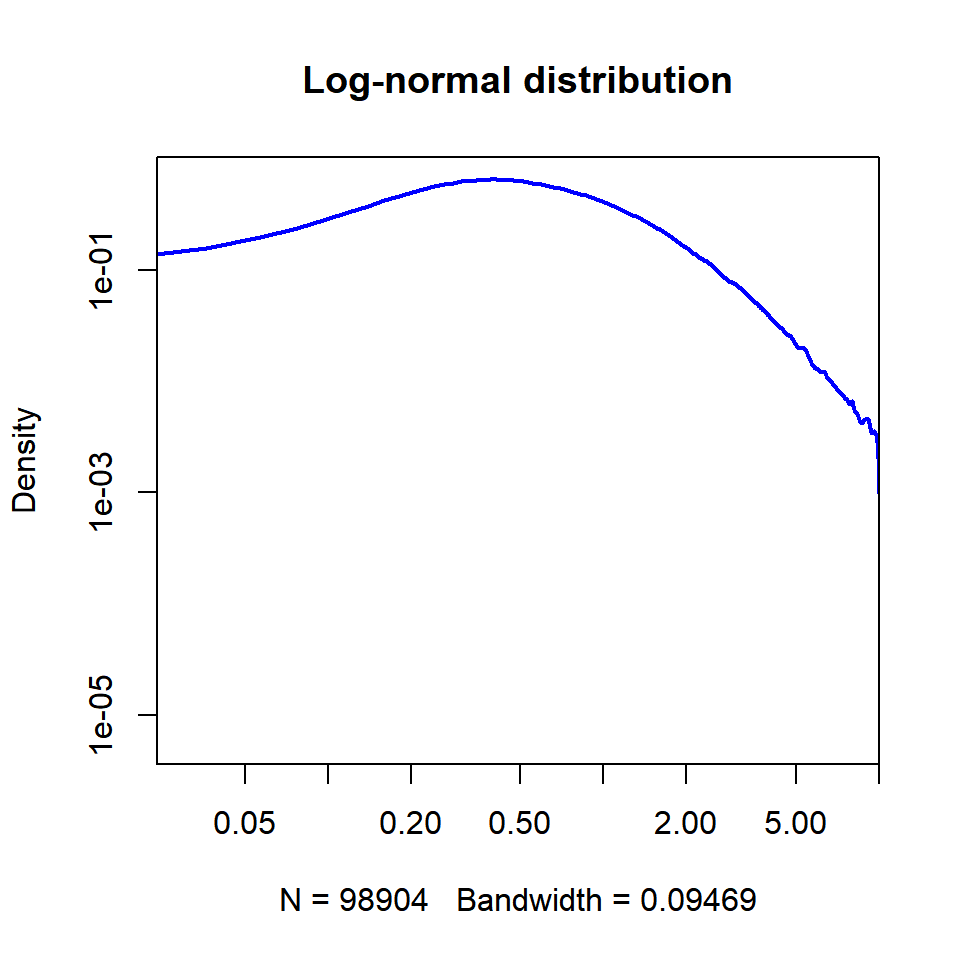which in the downsloping part can simulate a line as in the power law plotted in log-log.

Actually the lognormal plotted in log-log form is linear when the $$\sigma$$ value is high:

set.seed(1)
x = rlnorm(1e5, 0, 10)
x = x[x < 10]
plot(density(x), log="xy", type="l", xlim=c(.03, 8), col = 4, lwd = 2,
main="Log-normal distribution")